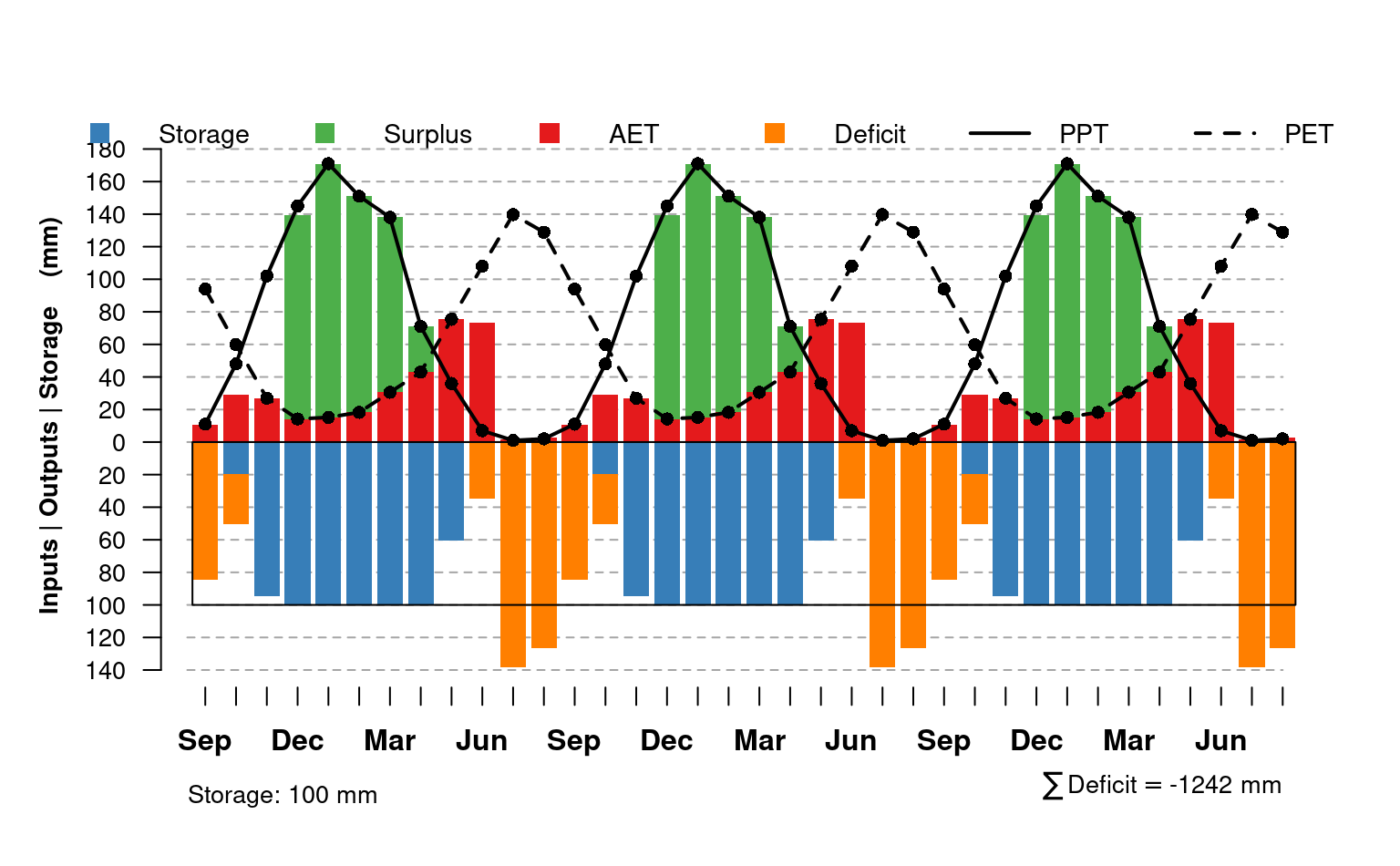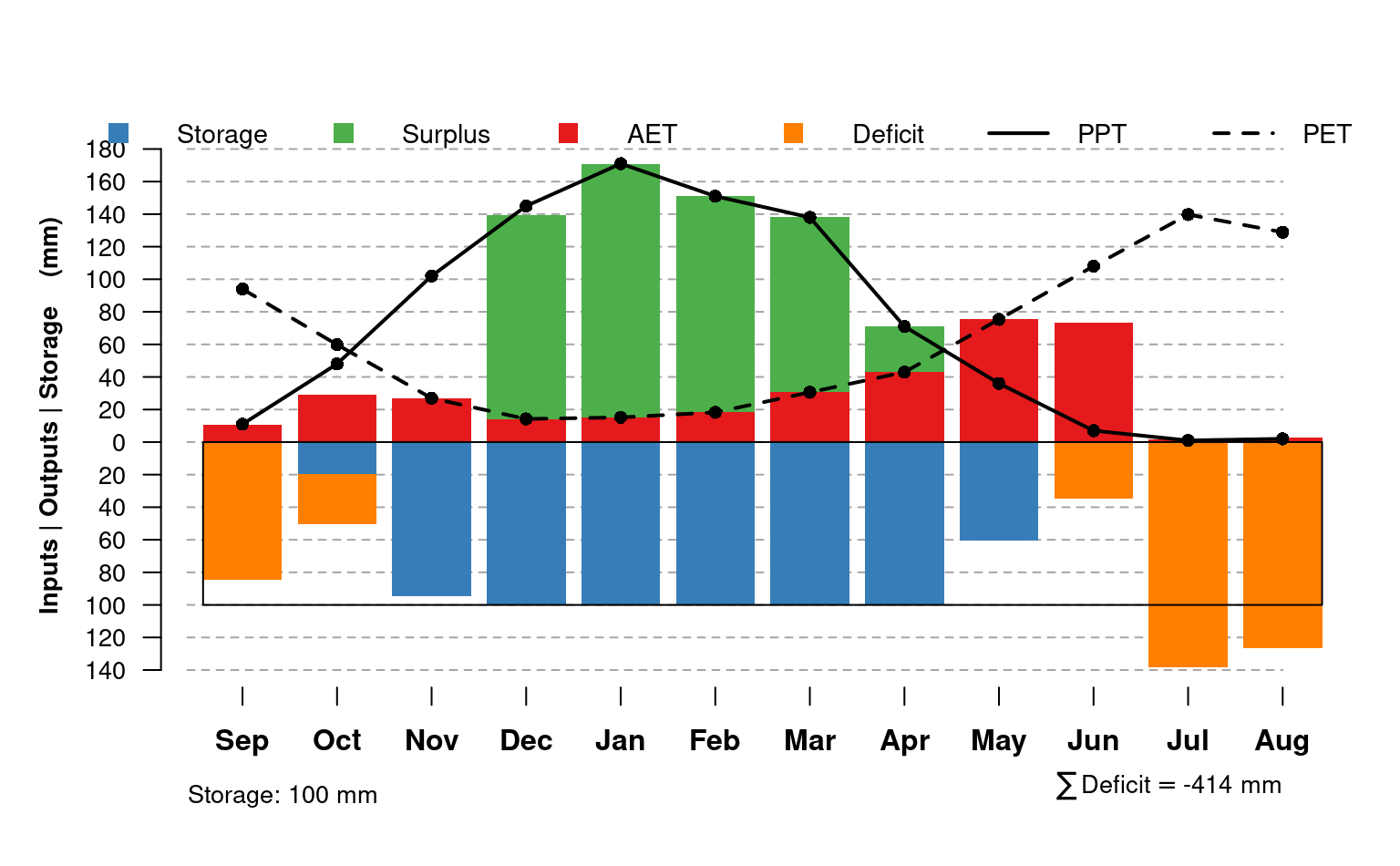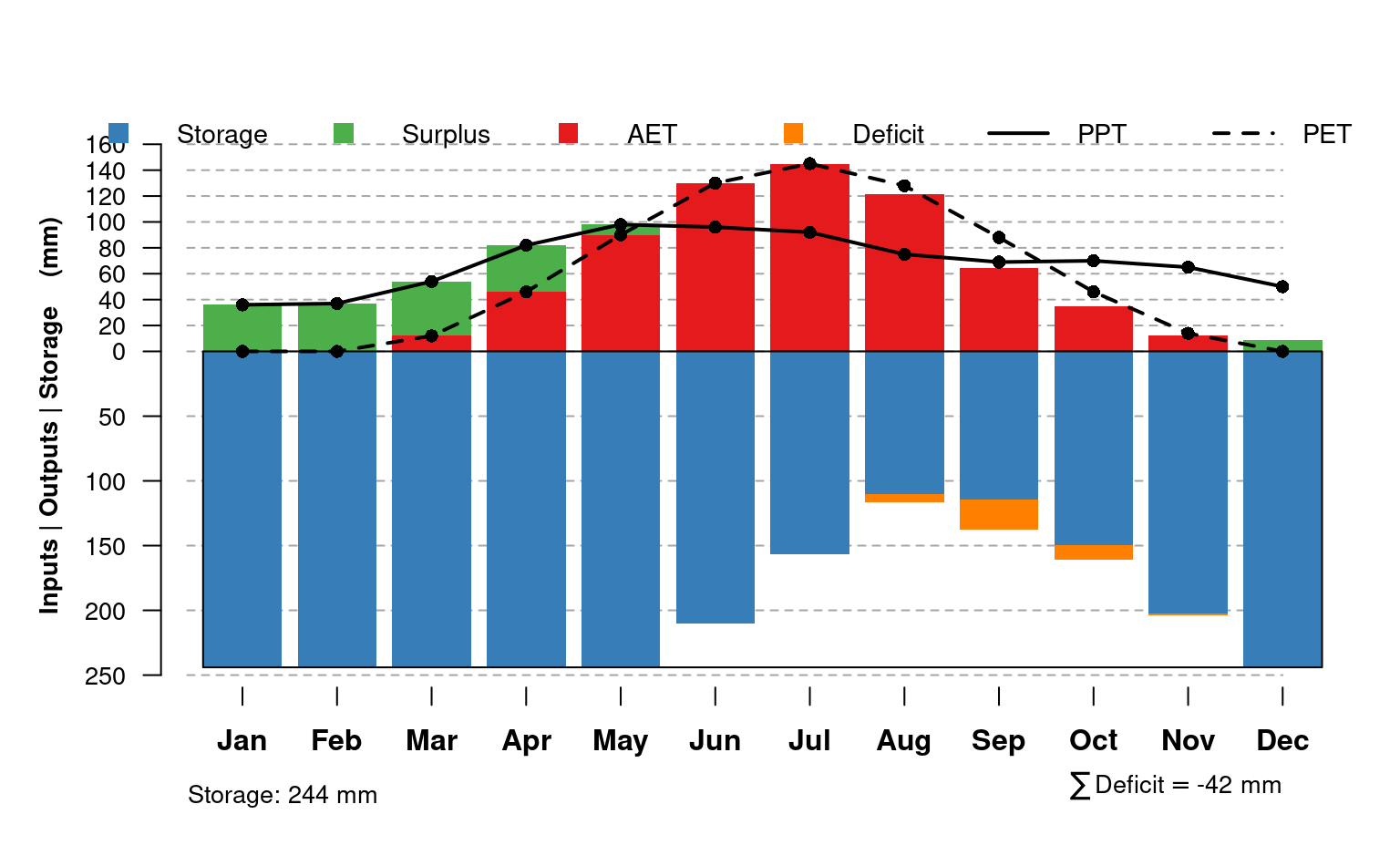This function offers one possible visualization for the results of monthlyWB(). Note that "surplus" water is stacked on top of "actual ET", and "deficit" water is stacked below "storage". Calculate actual values for "surplus" and "deficit" from the figure like this:

• surplus value = surplus - AET

• deficit value = deficit - storage

plotWB(
WB,
AWC = attr(WB, "AWC"),
sw.col = "#377EB8",
surplus.col = "#4DAF4A",
et.col = "#E41A1C",
deficit.col = "#FF7F00",
pch = c("P", "E"),
pt.cex = 0.85,
lwd = 2,
n.ticks = 8,
grid.col = grey(0.65),
month.cex = 1,
legend.cex = 0.9
)

## Arguments

WB

output from monthlyWB()

AWC

available water-holding capacity (mm), typically the value used in monthlyWB() and stored as an attribute of WB

sw.col

color for soil water ("storage)

surplus.col

color for surplus water

et.col

color for ET

deficit.col

color for deficit

pch

plotting character for PPT and PET points (c('P', 'E'))

pt.cex

character expansion factor for PPT and PET points

lwd

line width for PPT and PET curves

n.ticks

approximate number of tick marks on positive and negative y-axis

grid.col

horizontal grid line color

month.cex

scaling factor for month labels (x-axis)

legend.cex

scaling factor for legend

## Value

nothing, function is called to generate graphical output

## Note

You may have to adjust figure margins and size to get all of the elements to "look right".

## Author

D.E. Beaudette and J.M. Skovlin

## Examples



## A shallow / droughty soil near Sonora CA
# 100mm (4") AWC
AWC <- 100
PPT <- c(171, 151, 138, 71, 36, 7, 1, 2, 11, 48, 102, 145)
PET <- c(15.17, 18.26, 30.57, 42.95, 75.37, 108.05, 139.74, 128.9, 93.99, 59.84, 26.95, 14.2)

# water-year
# three years
x.wb <- monthlyWB(AWC, PPT, PET, S_init = 0, starting_month = 9, rep = 3)
x.wb[x.wb\$mo == 'Sep', ]

# plot all three years
plotWB(x.wb)

# water-year / last iteration
x.wb <- monthlyWB(AWC, PPT, PET, S_init = 0,
starting_month = 9, rep = 3,
keep_last = TRUE
)

# plot
plotWB(x.wb)

## Drummer series (Fine-silty, mixed, superactive, mesic Typic Endoaquolls), southern IL

AWC <- 244
PPT <- c(36, 37, 54, 82, 98, 96, 92, 75, 69, 70, 65, 50)
PET <- c(0, 0, 12, 46, 90, 130, 145, 128, 88, 46, 14, 0)

# using calendar year
x.wb <- monthlyWB(AWC, PPT, PET, S_init = 0,
starting_month = 1, rep = 3,
keep_last = TRUE
)

plotWB(x.wb)

}